## 4.1 Definitions, symbols and abbreviations

06.943GPPTSVoice Activity Detector (VAD) for Adaptive Multi Rate (AMR) speech traffic channels

### 4.1.1 Definitions

For the purposes of the present document, the following definitions apply:

codec: The combination of an encoder and decoder in series (encoder/decoder).

compand: The process of compressing and expanding a signal. In this text, the process is described in terms of PCM .

Decoder: Generally, a device for the translation of a signal from a digital representation into an analog format. For the present document, a device which converts speech encoded in the format specified in the present document to analog or an equivalent PCM representation.

DFT: See Discrete Fourier Transform.

Discrete Fourier Transform (DFT): A method of transforming a time domain sequence into a corresponding frequency domain sequence.

Encoder: Generally, a device for the translation of a signal into a digital representation. For the present document, a device which converts speech from an analog or its equivalent PCM representation to the digital representation described in the present document.

Fast Fourier Transform (FFT): An efficient implementation of the Discrete Fourier Transform.

FFT: See Fast Fourier Transform.

Vocoder: Voice coder.

frame: Time interval of 20 ms corresponding to the time segmentation of the speech transcoder.

### 4.1.2 Symbols

For the purposes of the present document, the following symbols apply.

#### 4.1.2.1 Variables

ch(m) channel energy smoothing factor

(m) exponential windowing factor

(m) estimated spectral deviation between current power spectrum and average long term power spectral estimate

(m) spectral peak-to-average ratio

q(i) quantized channel SNR indices

b(m) burst count

bth burst count threshold

{d(m)} overlapped portion of the frame buffer of input samples

Ech(m,i) channel energy estimate; channel i, subframe m

Ech(m) vector of channel energy estimates, 0  i < Nc

EdB(m,i) estimated log power spectrum

EdB(m) vector of log power spectrum estimates, 0  i < Nc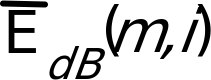average long term power spectral estimate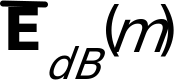vector of average long term power spectral estimates, 0  i < Nc

En(m,i) channel noise estimate

En(m) vector of channel noise estimates, 0  i < Nc

Etn(m) total estimated noise energy

Etot(m) total channel energy

Etot(m) modified total channel energy

h(m) hysteresis counter

hcnt hangover count

hyster_cnt hysteresis counter to avoid long term creeping of update_cnt

last_update_cnt previous value of update_cnt

shp(n) sample at the output of the speech encoder high pass filter

sinewave_flag boolean flag, set TRUE when spectral peak-to-average ratio is greater than 10dB and the spectral deviation is less than DEV_THLD

SNR Signal to Noise ratio

SNRp(m) long-term peak SNR

SNRq(m) quantized version of SNRp(m)

update_cnt counter gating noise estimate update process

update_flag flag controlling noise estimate updating

v(m) sum of voice metrics

vth voice metric threshold

#### 4.1.2.2 Constants

H upper limit for values of (m)

L lower limit for values of (m)

n channel noise smoothing factor

p pre-emphasis factor

btable table to generate bth

D overlap (delay) in sample intervals

DEV_THLD threshold for setting sinewave_flag

Efloor low threshold for Etot(m)

EH high energy endpoint for linear interpolation of Etot(m)

Einit minimum allowable channel noise initialisation energy

EL low energy endpoint for linear interpolation of Etot(m)

Emin minimum allowable channel energy

fH high channel combining table

fL low channel combining table

g(n) trapezoidal window, n = 0 to M

G(k) frequency domain transformation of g(n)

htable table to generate hcnt

HYSTER_CNT_THLD threshold for hyster_cnt

L subframe length in samples

M DFT sequence length

Nc number of combined channels

NOISE_FLOOR_D low threshold for Etot(m) in dB

UPDATE_CNT_THLD threshold for update_cnt

UPDATE_THLD threshold for v(m)

V voice metric table

vtable table to generate vth

#### 4.1.2.3 Functions

subtraction

* multiplication

/ division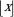largest integer  x

AND Boolean AND

OR Boolean OR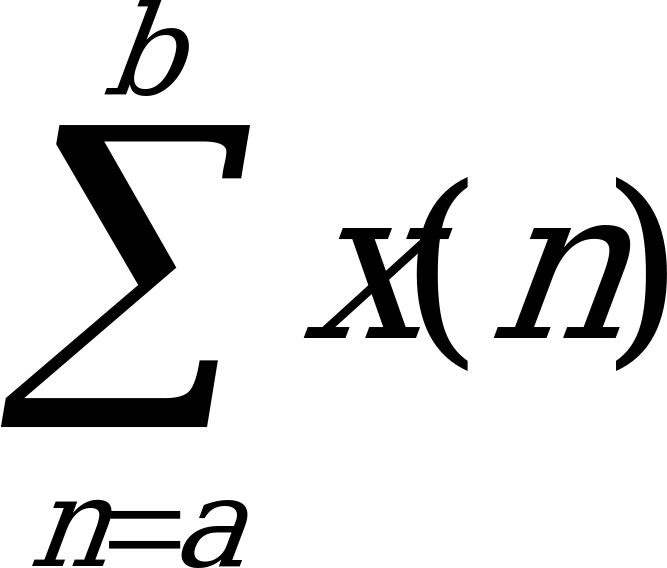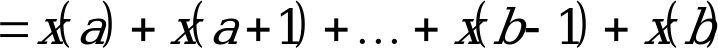### 4.1.3 Abbreviations

ANSI American National Standards Institute

DTX Discontinuous Transmission